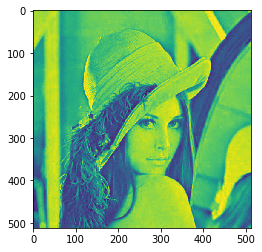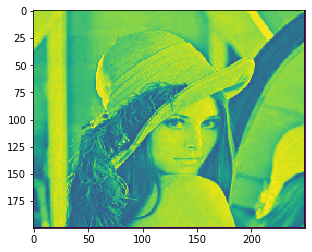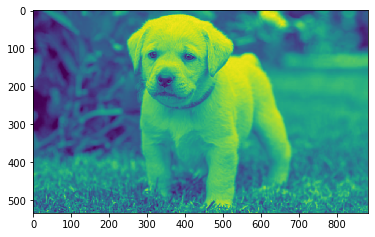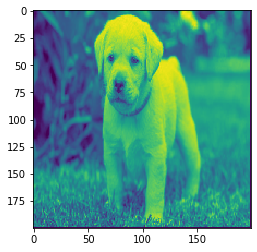Open In App

# Mahotas – Resizing Image

In this article we will see how we can resize the image in mahotas. Image interpolation occurs when you resize or distort your image from one pixel grid to another. Image resizing is necessary when you need to increase or decrease the total number of pixels, whereas remapping can occur when you are correcting for lens distortion or rotating an image.
In this tutorial we will use “lena” image, below is the command to load it.

`mahotas.demos.load('lena')`

Below is the lena imageIn order to do this we will use mahotas.imresize method
Syntax : mahotas.imresize(img, size)
Argument : It takes image object and new size as argument
Return : It returns image object

Note : Input image should be filtered or should be loaded as grey
In order to filter the image we will take the image object which is numpy.ndarray and filter it with the help of indexing, below is the command to do this

`image = image[:, :, 0]`

Below is the implementation

## Python3

 `# importing required libraries``import` `mahotas``import` `mahotas.demos``from` `pylab ``import` `gray, imshow, show``import` `numpy as np``import` `matplotlib.pyplot as plt``  ` `# loading image``img ``=` `mahotas.demos.load(``'lena'``)`  `  ` `# filtering image``img ``=` `img.``max``(``2``)` `print``(``"Image"``)``  ` `# showing image``imshow(img)``show()` `# resizing image``new_img ``=` `mahotas.imresize(img, [``200``, ``250``])`` `  `# showing image``print``(``"Resized Image"``)``imshow(new_img)``show()`

Output :

`Image``Resized Image`Another example

## Python3

 `# importing required libraries``import` `mahotas``import` `numpy as np``from` `pylab ``import` `gray, imshow, show``import` `os``import` `matplotlib.pyplot as plt`` ` `# loading image``img ``=` `mahotas.imread(``'dog_image.png'``)`  `# filtering image``img ``=` `img[:, :, ``0``]``  ` `print``(``"Image"``)``  ` `# showing image``imshow(img)``show()` `# resizing image``new_img ``=` `mahotas.imresize(img, [``200``, ``200``])`` `  `# showing image``print``(``"Resized Image"``)``imshow(new_img)``show()`

Output :

`Image``Resized Image`